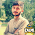## Wednesday, April 3, 2013

### creating grouped box plot in Excel (using RExcel)

See the related posts on RExcel (for basic, Excel 2003 and Excel 2007) for basic information.

In previous posts we discussed about RExcel getting started, here is one example of implementation - on creating grouped bar plots.

Just for example, we will use already available data in lattice package. This excercise will also demostrate how you can load data from R library and visualized in excel.

Let's use the data barley from R library (lattice package). I put data in excel sheet using Data ->  read data data set in packages, package datasets and clicking barley dataset .

In excel sheet click get active dataframe. The you can see the data embedded in the excel sheet.

In this dataset we different varities grown in different sites in different years. The measured variable is yield. Let's produced plotts in different ways. Let's summarize the data in different way one way is to create boxplot and compare over sites or years etc.

From graphs menu click boxplot and then select variable (yield) and a grouping variable (site in this example).

Here are other grouping outputs (by year, by variety) you want to plot.

Happy plotting !!

#### 1 comment:

1.how to create a clustered or grouped box plot in XLSTAT?